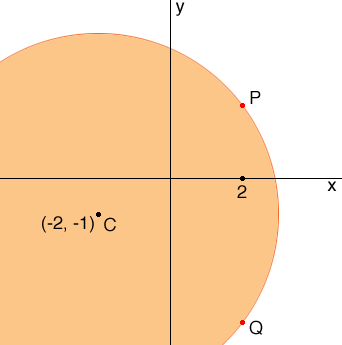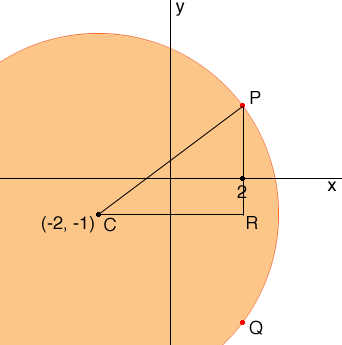SEARCH HOMEMath Central Quandaries & QueriesQuestion from Alexa, a student: I know the answer to the question, (I looked it up in the back of the book) but I need to know how to get the answer. Problem: Find all points having a x-coordinate of 2 whose distance from the point (-2, -1) is 5.Hi Alexa,

In my mind I see a diagram. The points in the plane that are 5 units from (-2, -1) make up the circle with center (-2, -1) and radius 5. You want the points on this circle with x-coordinate 2, the points labeled P and Q in the diagram.I then added a point R to complete the right triangle PCR.What does Pythagoras tell you?

Write back if you need more assistance,
PennyMath Central is supported by the University of Regina and The Pacific Institute for the Mathematical Sciences.